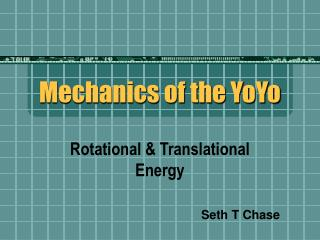DownloadDownload PresentationMechanics of the YoYo

# Mechanics of the YoYo

Télécharger la présentation## Mechanics of the YoYo

- - - - - - - - - - - - - - - - - - - - - - - - - - - E N D - - - - - - - - - - - - - - - - - - - - - - - - - - -
##### Presentation Transcript

1. Mechanics of the YoYo Rotational & Translational Energy Seth T Chase

2. Understand Energy in Motion • Energy of any particle is constant • Kinetic (T) + Potential (U) = Constant (E) • U = mgh • K = 2mv2 + 2Iω2 • I = 2mr2ω2 • E = mgh + 2mv2 + 3mr2ω2

3. Goals • Derive formula translational acceleration • Apply Euler algorithm to create relationships of motion and apply Leapfrog algorithm method to update visual • Relate rotational motion to derived formula for translational motion • Show energy is conserved by calculating forces within code

4. Derivation of Energy & Motion

5. Euler & Leapfrog Algorithm Creating Motion due to Gravity yoyoAccCM = ((2.0/3.0)*9.81) yoyo.acceleration = yoyoAccCM * vector(0,-1,0) yoyo.velocity.y = yoyo.velocity.y + yoyo.acceleration.y*dt yoyo.pos = yoyo.pos + yoyo.velocity*dt + yoyo.acceleration*dt*dt*0.5 Changing Direction yoyo.velocity.y = yoyo.omega*yoyo.radius_string yoyo.velocity.y = -yoyo.velocity.y - yoyo.acceleration.y*dt

6. Translational & Rotational Determining Angular Velocity (ω) yoyo.omega =(yoyo.velocity.y/yoyo.radius_string) w = yoyo.omega*direction Rotating Frame on Changing Position ypos = yoyo.pos.y yoyo.rotate(angle=(radians(w)), axis=(1,0,0), origin=(0,ypos,0))

7. Calculating Forces Total Energy Energy = (Potential + Kinetic) -(Energy*dt) Kinetic Energy K1 = Kinetic Kinetic =(.5*yoyo.mass*yoyo.velocity.y**2)+ (.25*yoyo.mass*(yoyo.omega*yoyo.radius_spin)**2) K2 = Kinetic Potential Energy P1=Potential Potential = -(yoyo.mass*yoyoGravCM*(8.8-yoyo.pos.y+ changeINposition)) P2=Potential

8. Additional Remarks on Code • Introduction to frames • Scenes and there benefits • Adding visual components of Energy Conservation

10. Creating a Scene vs. Conditional statements Creating Scene scene.width = 1000 scene.height = 1000 scene.center = (0,5.5,0) Creating Objects with Variable Components mass1 = box(pos=(3.5,12.5,.2) ,length=.6, height=.6, width=.6,color=color.red) mass1.mass = .005 message = "Click to add 1.0 gram." mass1Label = label(pos=(4,12.3,.2), text=message,xoffset=20) Detecting and Implementing Mouse Click if scene.mouse.events: # detect mouse events mouse = scene.mouse.getclick() if mouse.pick == mass1 or mouse.pick==mass2: right_side.radius += mouse.pick.mass

11. Sliding Bars of Kinetic & Potential Energy Creating Boxes and Bars PBar = box(pos=(5.5,6.65,.1), length=.9, height=.1, width=.9) PBox=box(pos=(5.5,6.465,0),length=1, height=1.34, width=1, color=color.red) message = "Potential Energy" PboxLabel = label(pos=(4.9,6.7,0),text=message,xoffset=-17) Updating Bars to Move in Box PBar.pos.y = (PBox.pos.y+(PBox.height/2)-(PBar.height/2))- ((PBox.height*(P2/P_mag))/(2*handle.pos.y))

12. Problems within Code • Bouncing at same height • Eliminating errors by increasing rate and decreasing dt • Finding right proportions of position change when increasing mass and showing via thickness

13. Conservation of Energy

14. Calculating Drag

15. Effects of Drag

16. Drag Force with Increasing Temperature over Time